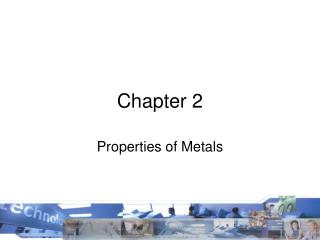DownloadDownload PresentationChapter 2

# Chapter 2

Télécharger la présentation## Chapter 2

- - - - - - - - - - - - - - - - - - - - - - - - - - - E N D - - - - - - - - - - - - - - - - - - - - - - - - - - -
##### Presentation Transcript

1. Chapter 2 Properties of Metals

2. Stress-Strain Curve Figure 2.2 A typical stress- strain curve obtained from a tension test, showing various features.

3. Strain- • The unit deformation of a material when stress is applied.

4. Stress- • The load per unit of area on a stress-strain diagram.

5. (b) Tensile-Test Specimen and Machine Figure 2.1 (a) A standard tensile-test specimen before and after pulling, showing original and final gage lengths. (b) A typical tensile-testing machine.

6. Tensile stress- • Refers to an object loaded in tension, denoting the longitudinal force that causes the fibers of a material to elongate.

7. Temperature Effects on Stress-Strain Curves Figure 2.7 Typical effects of temperature on stress-strain curves. Note that temperature affects the modulus of elasticity, the yield stress, the ultimate tensile strength, and the toughness (area under the curve) of materials.

8. Proportional limit- • On the stress-strain diagram, the point where the ratio of stress to strain (i.e., the modulus of elasticity) is no longer a constant, or where that line is no longer straight.

9. Stress-Strain Curve Figure 2.2 A typical stress- strain curve obtained from a tension test, showing various features.

10. Yield strength- • The stress at which a material deviates by a specified amount of strain from the region where stress and strain are proportional.

11. Ultimate tensile strength or UTS- • The stress equal to the maximum load achieved in a tensile test divided by the original area of the specimen.

12. Modulus of elasticity- • The ratio of the unit stress to the unit deformation (strain) of a structural material; a constant as long as the unit stress is below the elastic limit. • Also known as Young’s Modulus • Shearing modulus of elasticity is often called the modulus of rigidity.

13. Creep- • Slow plastic deformation in steel and most structural metals caused by prolonged stress under the yield point at elevated temperatures.

14. Compressive stress- • Refers to a member loaded in compression, which gives rise to either a given reduction in volume

15. Shear load- • A load that tends to force materials apart by application of side-slip action.

16. Tensile load- • A load applied to a part or parts that attempts to pull apart by a stretching action.

17. Ductility- • The property of a material that allows it to fail permanently, or to exhibit plasticity without rupture while under tension.

18. Impact test- • A test that applies an impact load (by a swinging hammer) to a small notched specimen. • The data from the test are in energy units, joules or inch- pounds. • The test is performed with the specimens at different temperatures; the results determine the notch toughness of the metals at the temperature tested. • There are two types of specimens- • Izod • Charpy, with Charpy the one primarily used in the United States.

19. Impact Test Specimens Figure 2.18 Impact test specimens: (a) Charpy; (b) Izod.

20. Malleability- • The ability of a metal to deform permanently without rupture when loaded in compression.

21. Fatigue in metals- • The tendency of a metal to fail by breaking or cracking under conditions of repeated cyclical stressing that takes place well below the ultimate tensile strength.

22. Hardening- • The process of increasing the hardness of a ferrous alloy by austenitizing and quenching; also, the process of increasing the hardness of some stainless steels and nonferrous alloys by solution heat treatment and precipitation.

23. Hardness- • The property of a metal that allows it to resist being permanently deformed. • This property is divided into three categories: • the resistance to penetration • the resistance to abrasion • elastic hardness.

24. Hardness Tests Figure 2.12 General characteristics of hardness-testing methods and formulas for calculating hardness. The quantity P is the load applied. Source: H. W. Hayden, et al., The Structure and Properties of Materials, Vol. III (John Wiley & Sons, 1965).

25. Plasticity- • The quality of material such that it can be deformed without breaking. • Clay is a completely plastic material. • Metals exhibit plasticity in varying amounts.